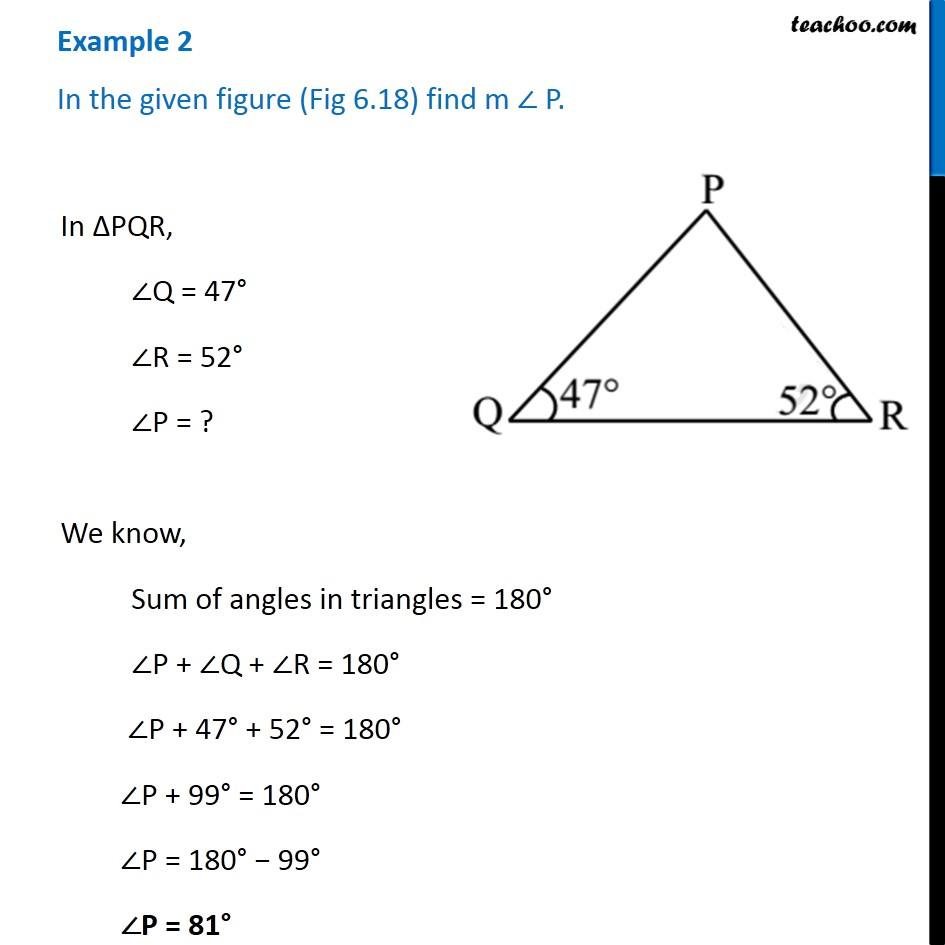Angle sum property of a triangle

Chapter 6 Class 7 Triangle and its Properties
Concept wiseIntroducing your new favourite teacher - Teachoo Black, at only ₹83 per month

### Transcript

Example 2 In the given figure (Fig 6.18) find m ∠ P. In ∆PQR, ∠Q = 47° ∠R = 52° ∠P = ? We know, Sum of angles in triangles = 180° ∠P + ∠Q + ∠R = 180° ∠P + 47° + 52° = 180° ∠P + 99° = 180° ∠P = 180° − 99° ∠P = 81°